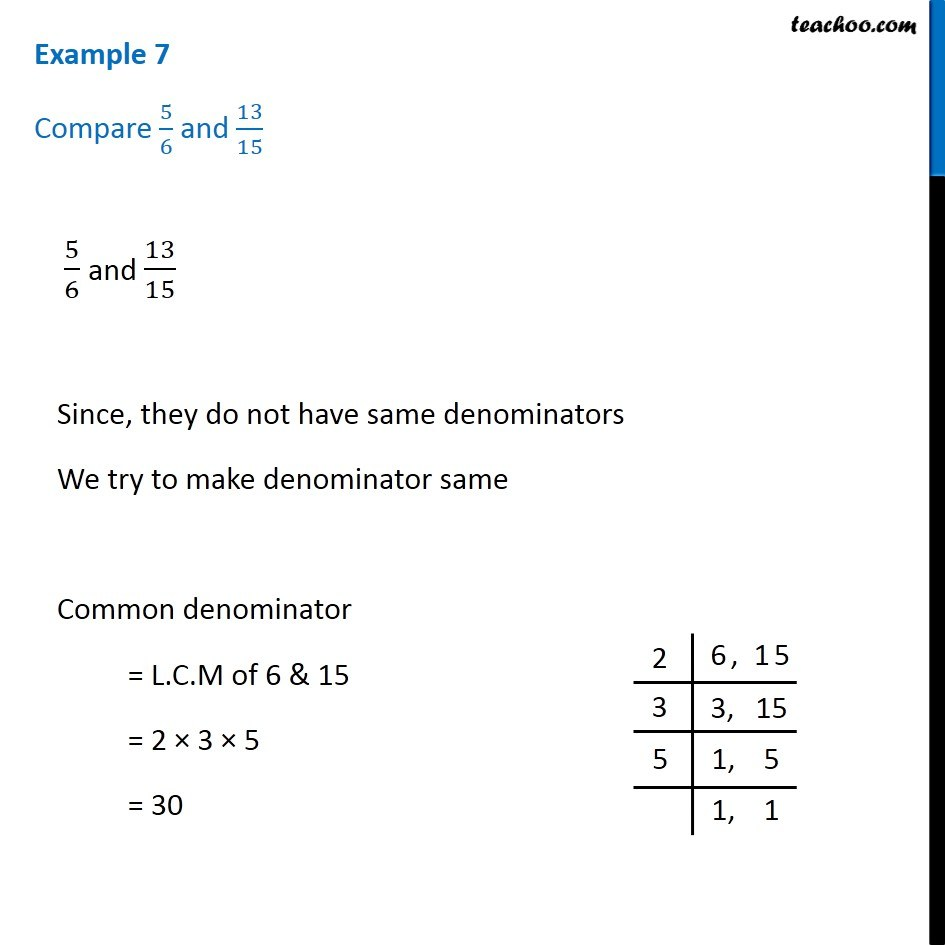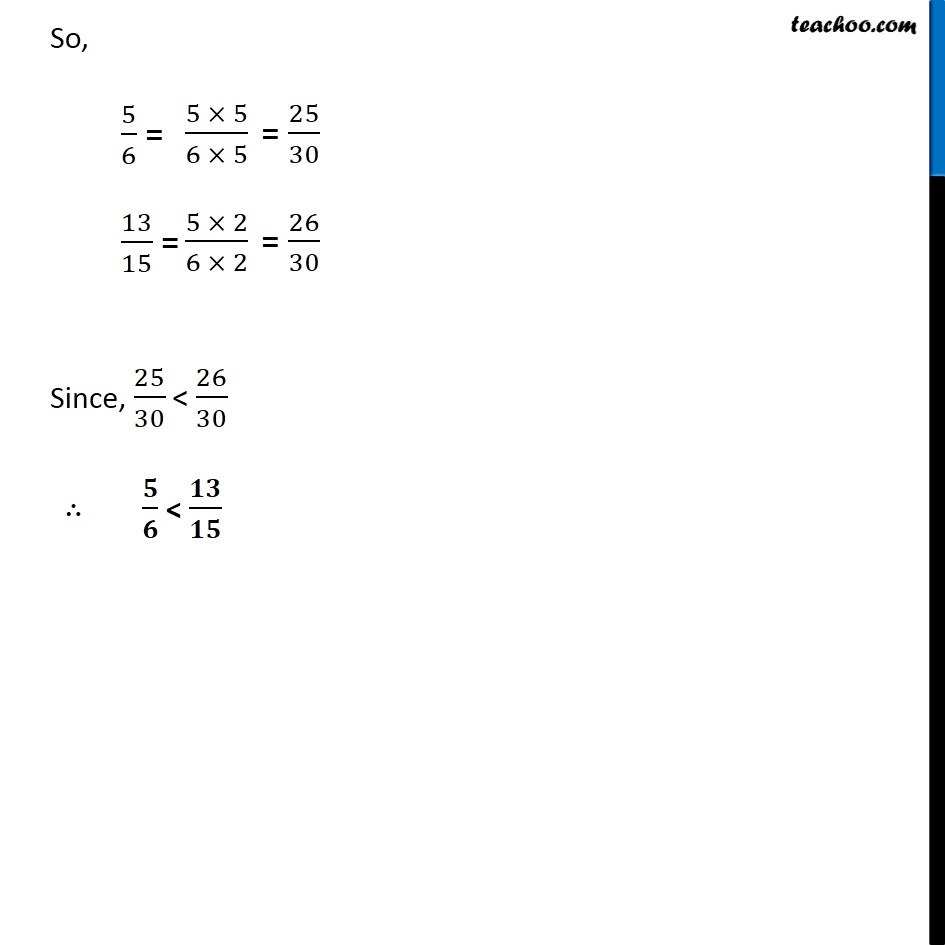1. Chapter 7 Class 6 Fractions
2. Serial order wise
3. Examples

Transcript

Example 7 Compare 5/6 and 13/15 5/6 and 13/15 Since, they do not have same denominators We try to make denominator same Common denominator = L.C.M of 6 & 15 = 2 × 3 × 5 = 30 So, 5/6 = 13/15 = Since, 25/30 < 26/30 ∴ 𝟓/𝟔 < 𝟏𝟑/𝟏𝟓

Examples

Chapter 7 Class 6 Fractions
Serial order wise